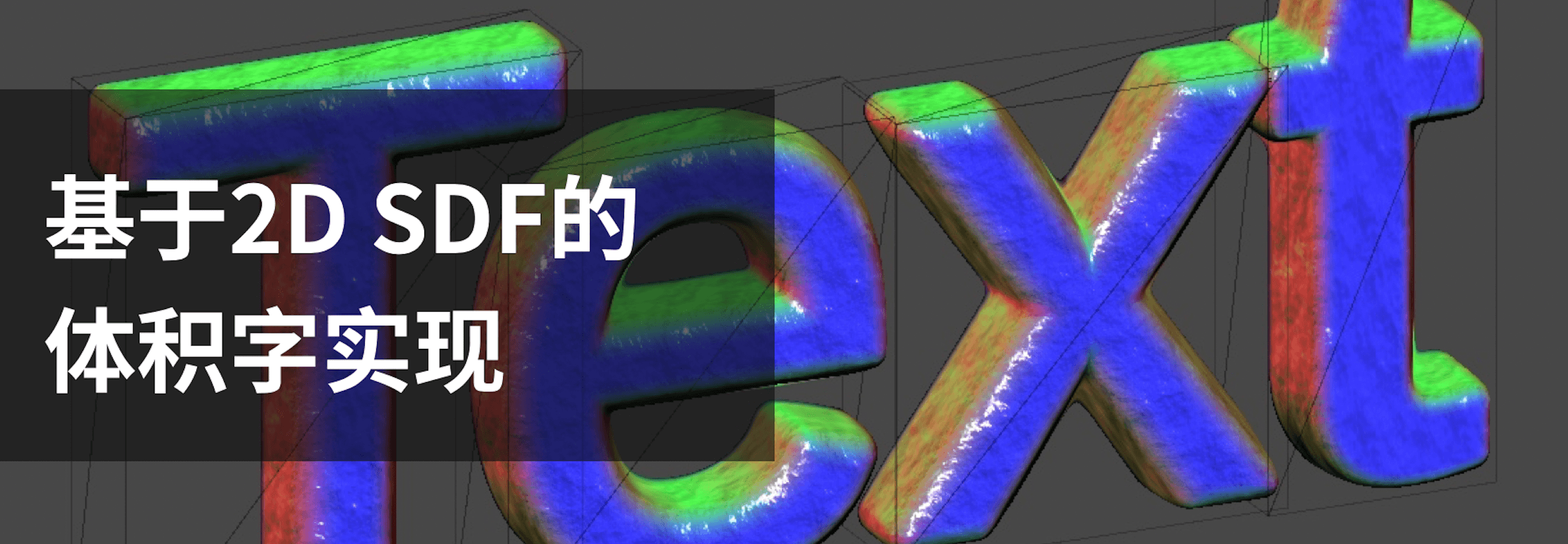# 基于2D SDF的体积字实现

## 概述

Volumetric Rendering - Alan Zucconi

Ray Marching and Signed Distance Functions

## 一、形状计算

1.1 SDF数据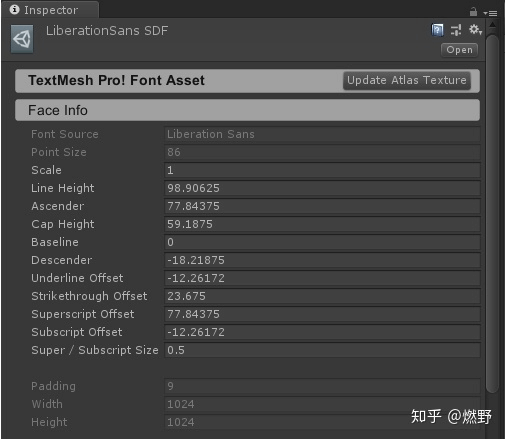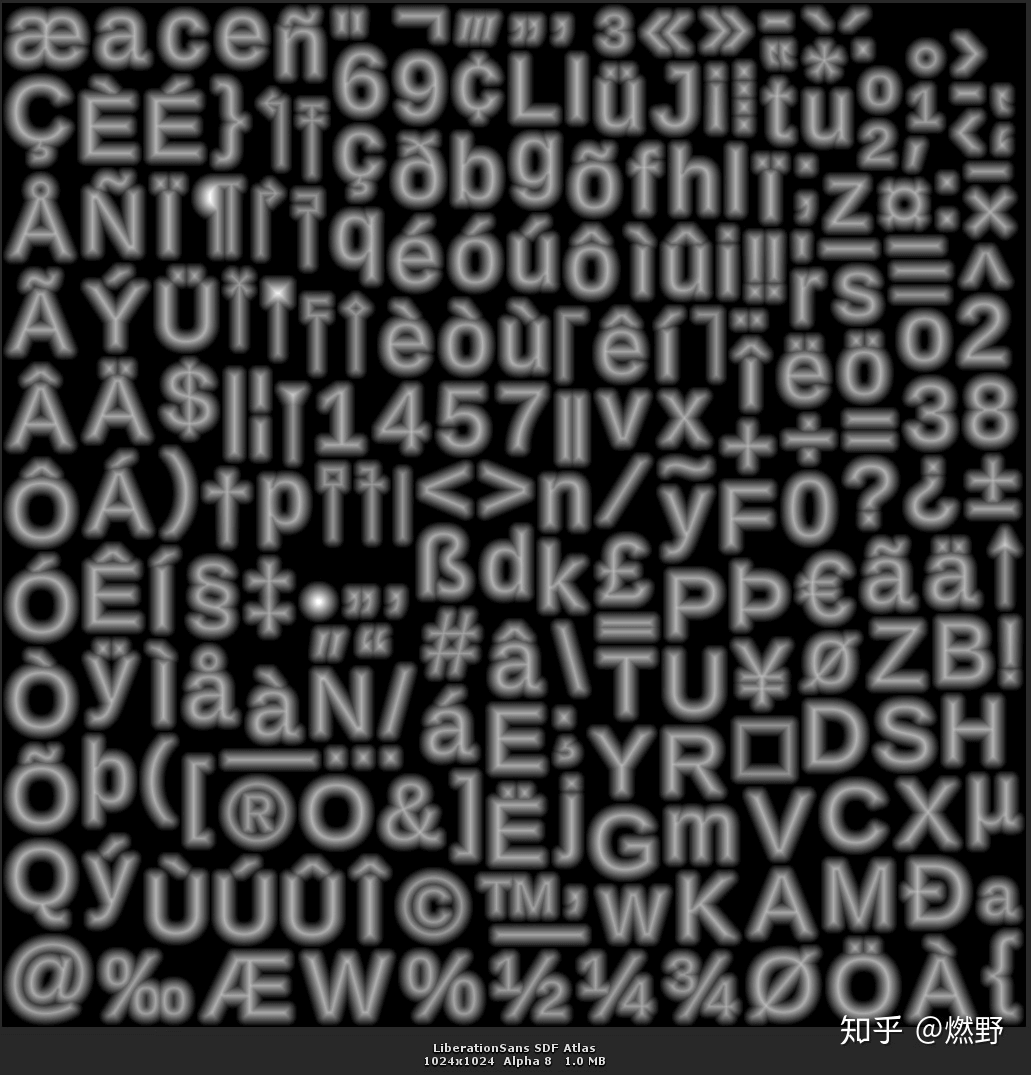1.2 体和坐标系

2D SDF拉出一个厚度能表示一个3D场，可以对应一个Cube模型，长宽高可以不相等，“显然”于模型内的任一点都能算出是否属于体积字之内。TMP生成的2D片局部坐标都是在XY平面上的，正面朝向负Z，见下图：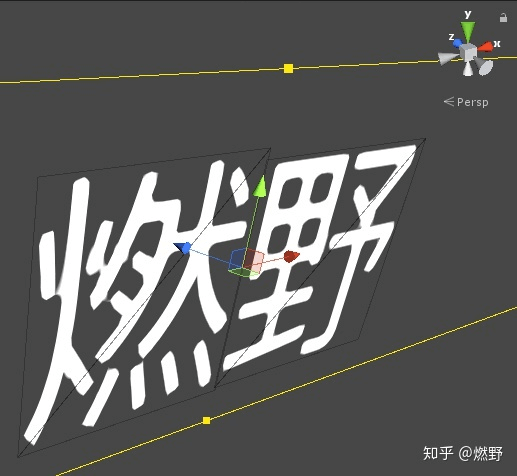1.3 大概算法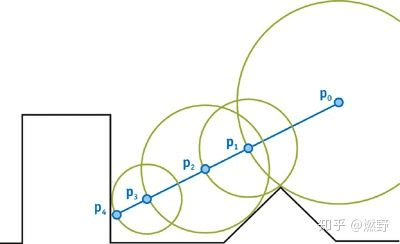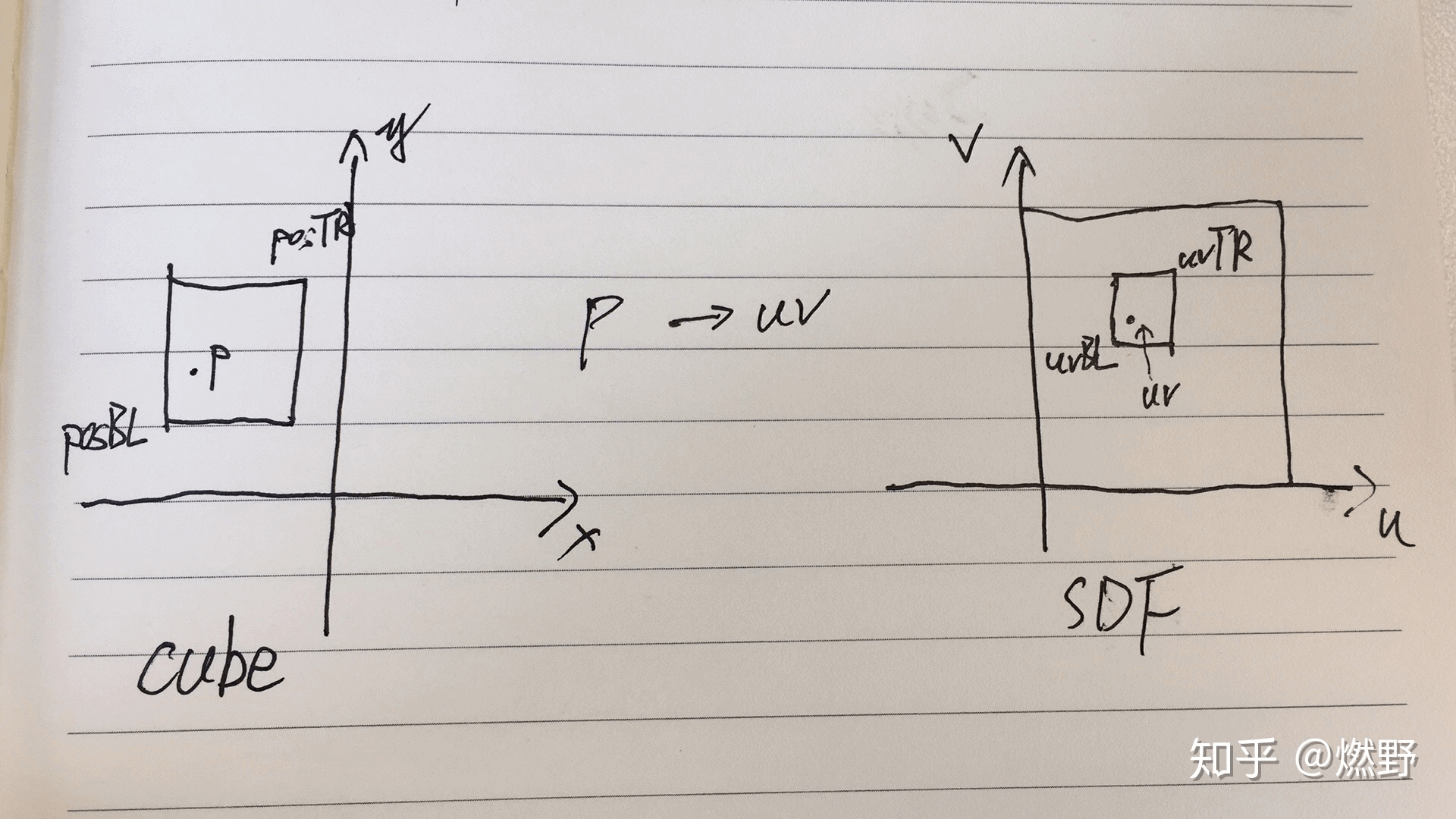sdf2len = 2 * _GradientScale / (max(_TextureWidth * sdfUvScale.x, _TextureHeight * sdfUvScale.y))

unpack posBLAndUvFactor，算出sdfUvScaleOffset ，给定字符局部坐标p，可以得到sdfUV = p.xy * sdfUvScaleOffset.xy + sdfUvScaleOffset.zw。FaceUV同理。

``````// UnityCG.cginc里的view dir相关方法
// Computes world space view direction, from object space position
inline float3 UnityWorldSpaceViewDir( in float3 worldPos )
{
return _WorldSpaceCameraPos.xyz - worldPos;
}

// Computes world space view direction, from object space position
inline float3 WorldSpaceViewDir( in float4 localPos )
{
float3 worldPos = mul(unity_ObjectToWorld, localPos).xyz;
return UnityWorldSpaceViewDir(worldPos);
}

// Computes object space view direction
inline float3 ObjSpaceViewDir( in float4 v )
{
float3 objSpaceCameraPos = mul(unity_WorldToObject, float4(_WorldSpaceCameraPos.xyz, 1)).xyz;
return objSpaceCameraPos - v.xyz;
}
``````

VS还是比较简单的，一个字符8个顶点，计算量也不大，PS里的重复计算可以移动到VS里来算。

``````// vs的输入
struct appdata
{
float4 vertex       : POSITION;
float3 bound        : NORMAL;   // 字符cube的长宽高
float4 posBLAndUvFactor     : TANGENT;  // xy是字符cube左下点的局部坐标，zw是sdf和face的uv转换系数被pack的值
fixed4 color        : COLOR;
float2 uv0              : TEXCOORD0;    // uvBL
float2 uv1              : TEXCOORD1;    // uv2BL
};

// ps的输入
struct v2f
{
float4 vertex                : SV_POSITION;
fixed4 faceColor             : COLOR;
float4 sdfUvScaleOffset      : TEXCOORD0;   //转换mesh局部坐标到sdf uv，xy是系数，zw是常量
float4 faceUvScaleOffset     : TEXCOORD1;   //转换mesh局部坐标到face uv，xy是系数，zw是常量
float3 viewDir               : TEXCOORD2;   //
float3 cuboidLocalPos        : TEXCOORD3;   //xyz是位置，左下角在原点。
float4 bound                 : TEXCOORD4;   // xyz是模型size, w是转换sdf距离到模型距离的系数
float3 localLightPos         : TEXCOORD5;   //字符局部坐标系下光的位置，表面到光源方向
float4 volParam              : TEXCOORD6;   //x是模型空间下的轮廓圆角半径
};

v2f Vert (appdata v)
{
v2f o;
o.vertex = UnityObjectToClipPos(v.vertex);
o.faceColor = v.color;

// 左下角平移到局部坐标系原点，此时坐标范围是[0, bound]
o.cuboidLocalPos = v.vertex - float4(v.posBLAndUvFactor.xy, 0, 0);

float2 inverseBound = 1 / v.bound.xy;
float2 sdfUvScale = UnpackFloat(v.posBLAndUvFactor.z, 1) * inverseBound / float2(_TextureWidth, _TextureHeight);
o.sdfUvScaleOffset = float4(sdfUvScale, v.uv0);

float2 faceUvScale = UnpackFloat(v.posBLAndUvFactor.w, 512) * inverseBound;
o.faceUvScaleOffset = float4(faceUvScale, UnpackFloat(v.uv1.x, 512));

// 视线转换到模型局部坐标系，此时是整个模型（很多字符）的局部坐标系，到ps里再normalize
// _WorldSpaceCameraPos是float3，补上w=1才能正确转换
// 没找到更好的计算透视和正交的方法，clip坐标系下载近平面的投影转换到localspace比较好，但没看到InvMVP矩阵
float3 perspectiveViewDir = v.vertex - mul(unity_WorldToObject, float4(_WorldSpaceCameraPos.xyz, 1));
float3 orthographicViewDir = mul(unity_WorldToObject, mul(unity_MatrixInvV, float4(0, 0, -1, 0)));
o.viewDir = lerp(perspectiveViewDir, orthographicViewDir, unity_OrthoParams.w);

// xy方向上可能是不一致的，为了正确性取相对小的步长
float sdf2len = 2 * _GradientScale / (max(_TextureWidth * sdfUvScale.x, _TextureHeight * sdfUvScale.y)) * _stepLenFactor;
o.bound = float4(v.bound, sdf2len);

o.localLightPos = mul(unity_WorldToObject, _WorldSpaceLightPos0);

o.volParam = float4(v.bound.x * _outlineRadius, 0, 0, 0);
return o;
``````

1.5 PS里算RayMarching

``````loat SampleSdfValueByLocalPos(v2f input, float3 localPos)
{
float2 sdfUv = localPos.xy * input.sdfUvScaleOffset.xy + input.sdfUvScaleOffset.zw;
// 编译警告说for里得用lod版本的tex2D
return tex2Dlod(_MainTex, float4(sdfUv, 0, 0)).a;
}

float2 RayMarching(v2f input, out float3 localPos, float3 viewDir)
{
localPos = input.cuboidLocalPos.xyz;

// 除以水平距离，这样在乘以水平距离就知道3d方向的增量
viewDir /= max(length(viewDir.xy), 0.001);

int i = 0;
float sdfValue;
for (; i < _loopCount; i++)
{
sdfValue = SampleSdfValueByLocalPos(input, localPos);
float sdfDist = _outline - sdfValue;
float meshDist;

if (sdfDist <= _outlineEpsilon)
return float2(i, sdfValue);

meshDist = sdfDist * input.bound.w;

// sdf距离转到模型距离，向前march
localPos += viewDir * meshDist;

// 到达模型外面要被剔除掉
// 剔除并不需要epsilon解决精度问题比较欣慰
clip(localPos * (input.bound.xyz - localPos));
}

// 没有到达范围内，但应该也很接近了
sdfValue = SampleSdfValueByLocalPos(input, localPos);
return float2(i, sdfValue);
}

// ps
fixed4 Frag (v2f input) : SV_Target
{
float3 localPos;
float3 viewDir = normalize(input.viewDir);
float2 result = RayMarching(input, localPos, viewDir);
fixed4 col = 1 - result.x / _loopCount;
col.a = 1;
return col;
}
``````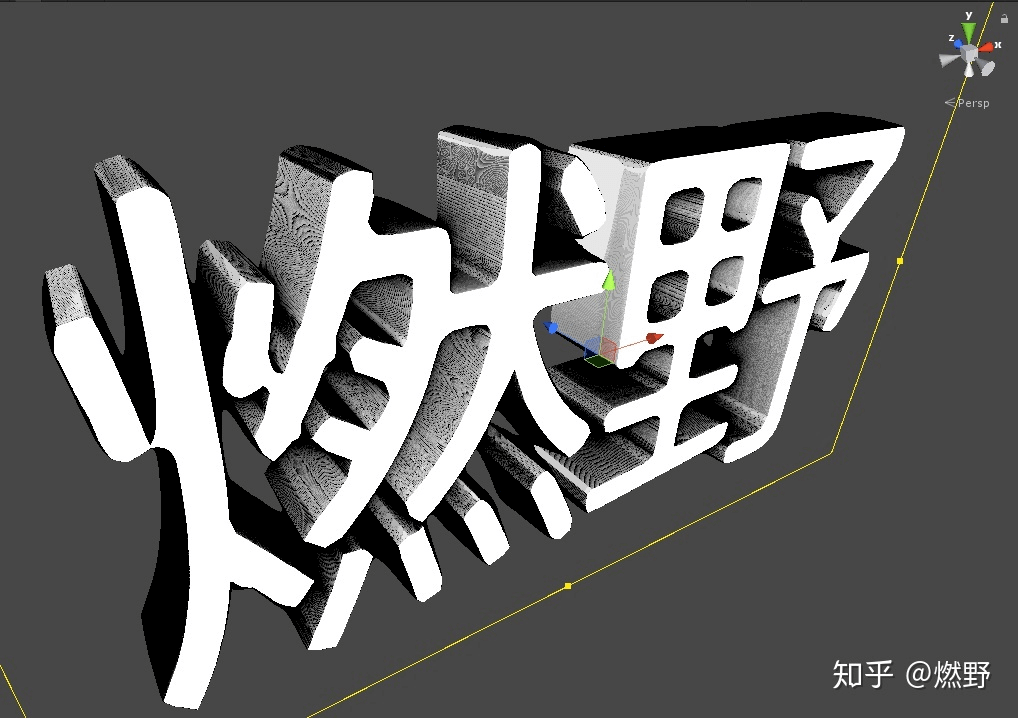_loopCount为10，_outlineEpsilon为0的情况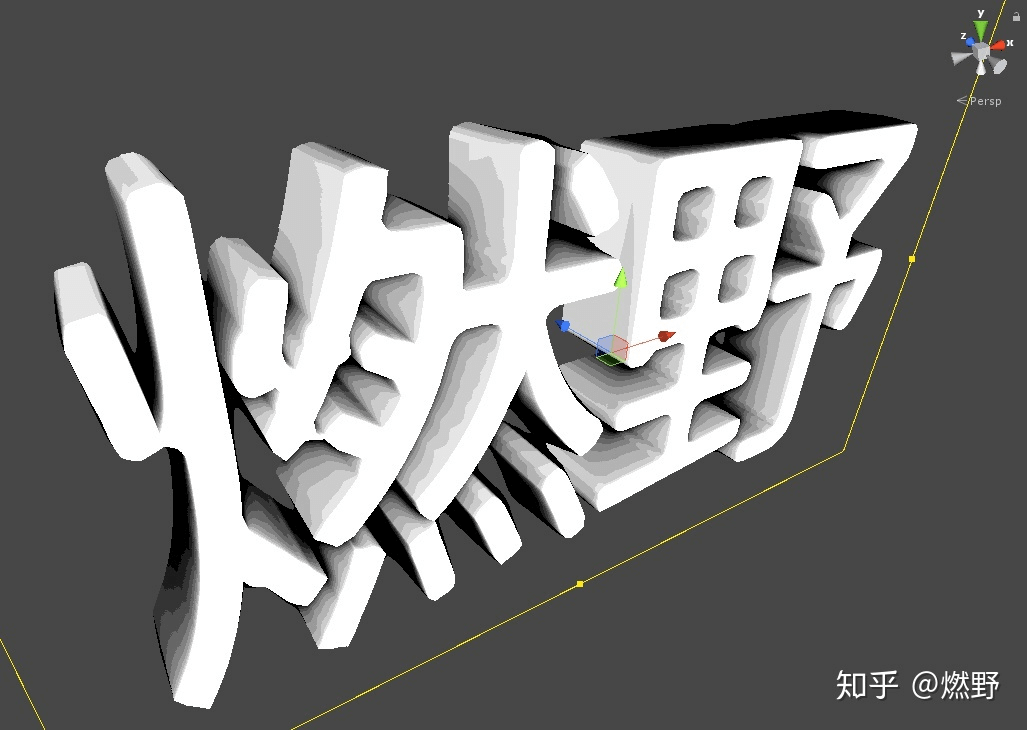_loopCount为10，_outlineEpsilon为0.01的情况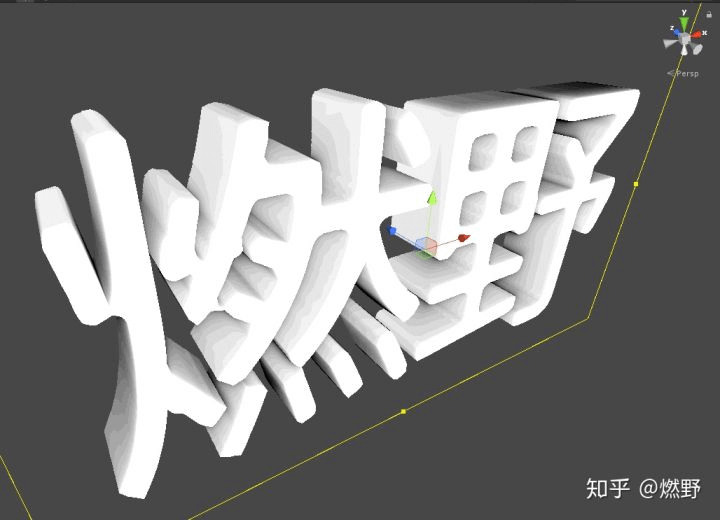_loopCount为20，_outlineEpsilon为0.01的情况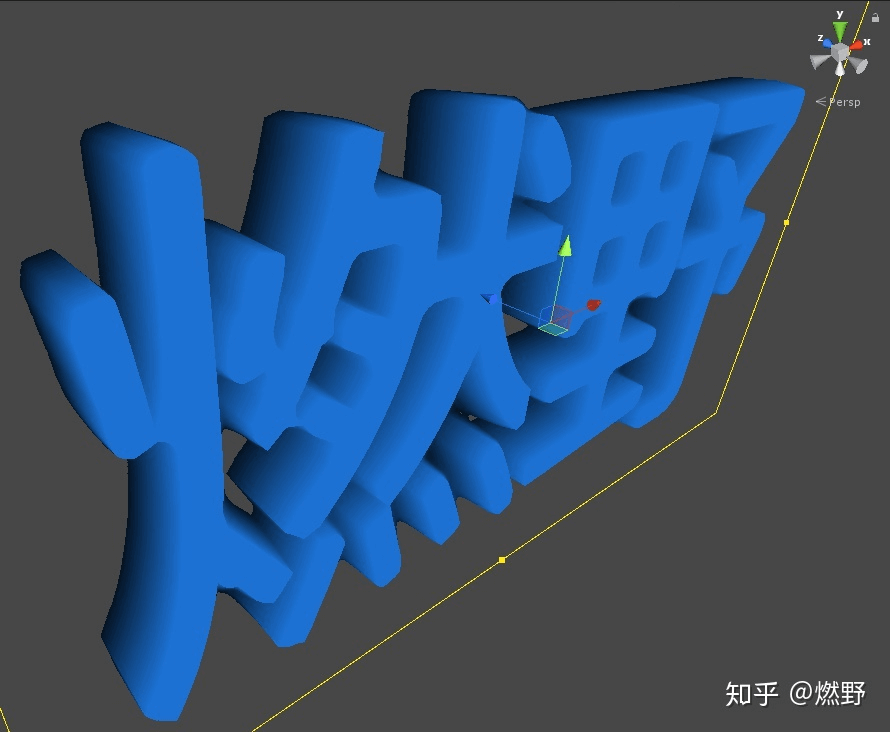_loopCount为10，_outlineEpsilon为0.01的情况

## 二、字体法线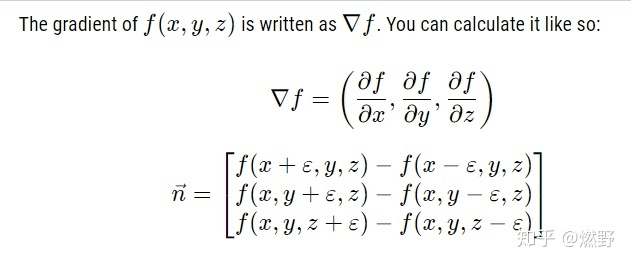z方向没变化为0，只算xy方向SDF值的梯度即可，_SideNormalSampleDelta用于微调计算梯度的Delta。

``````// 计算法线，前后面法线通过z计算，4个侧面法线是sdf的下降梯度
void ComputeNormal(v2f input, float3 localPos, out float3 frontBackNormal, out float3 sideNormal)
{
// 前或后面的法线，不是侧面的. 圆角过渡部分也需要正反面法线
frontBackNormal = float3(0, 0, localPos.z > input.bound.z - localPos.z ? 1 : -1);

// 用小位置差算梯度，得在中心点周围的4个点采样，只采样2个是不对的
float2 deltaPos = input.bound.xy * _SideNormalSampleDelta;
float sdfDeltaX1 = SampleSdfValueByLocalPos(input, localPos + float3(deltaPos.x, 0, 0));
float sdfDeltaX0 = SampleSdfValueByLocalPos(input, localPos - float3(deltaPos.x, 0, 0));
float sdfDeltaY1 = SampleSdfValueByLocalPos(input, localPos + float3(0, deltaPos.y, 0));
float sdfDeltaY0 = SampleSdfValueByLocalPos(input, localPos - float3(0, deltaPos.y, 0));
// 避免除以0，不然所在像素颜色未定义
sideNormal = Unity_SafeNormalize(float3(sdfDeltaX0 - sdfDeltaX1, sdfDeltaY0 - sdfDeltaY1, 0));
}

// z很接近前后面后frontBackNormal，否则用sideNormal，光照部分就不贴出来了
``````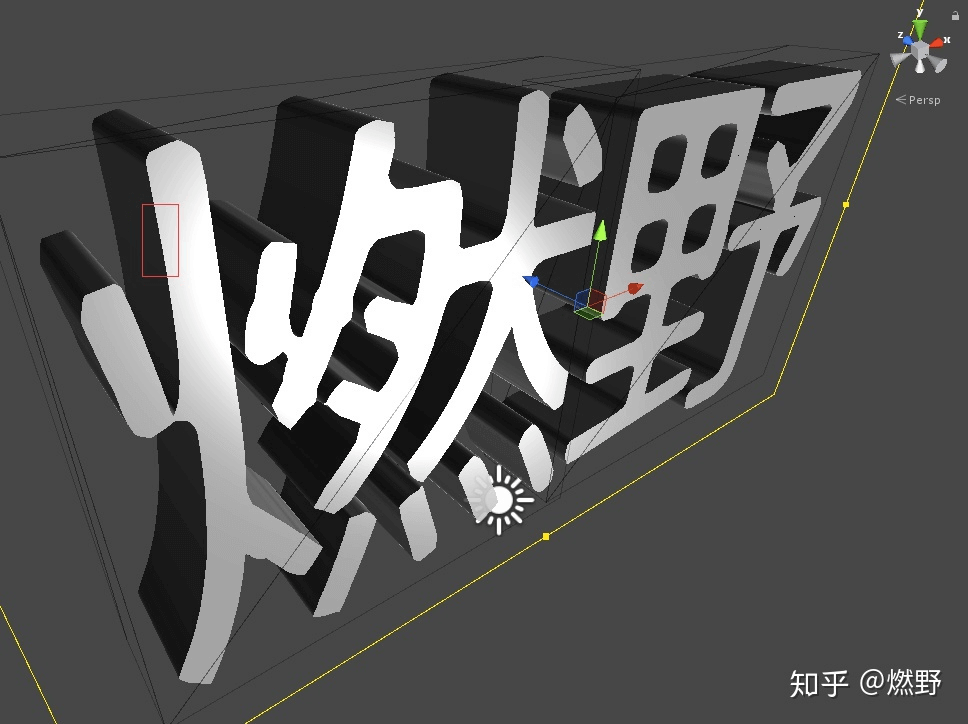2.1 字形法线的圆角过渡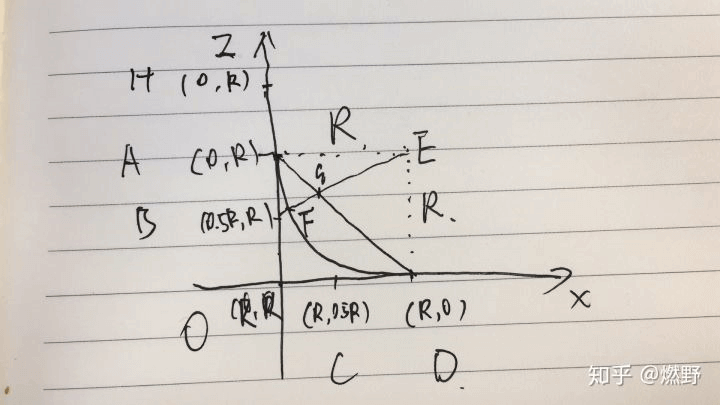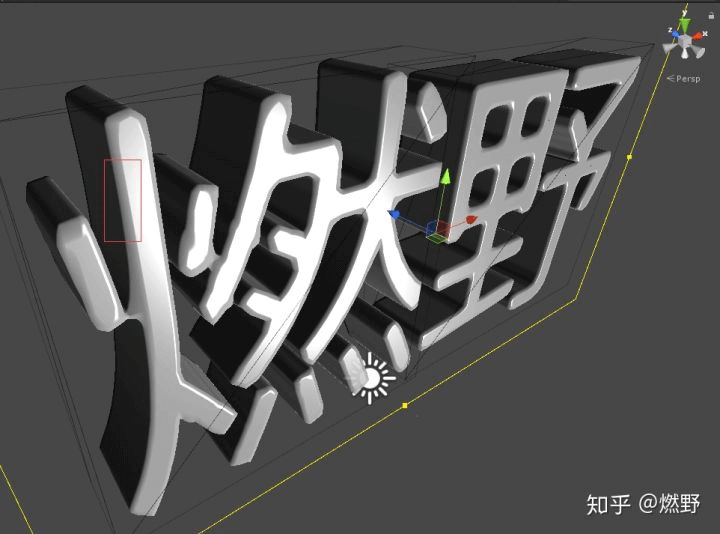``````// 计算圆角过渡中侧面的权重，input.volParam.x是半径
float ComputeSideWeight(v2f input, float3 localPos, float sdfValue)
{
// sdf中到边界的距离，转换为模型的长度
float toOutlineDist = (sdfValue - _outline) * input.bound.w;
float outlineFactor = max(input.volParam.x - toOutlineDist, 0);
float depthFactor = max(input.volParam.x - min(localPos.z, input.bound.z - localPos.z), 0);

// 修改斜率，使圆角边缘平缓，中间陡一点
outlineFactor *= outlineFactor;
depthFactor *= depthFactor;
// 对side和frontback做过渡
float sideWeight = outlineFactor / (outlineFactor + depthFactor);
return sideWeight;
}
``````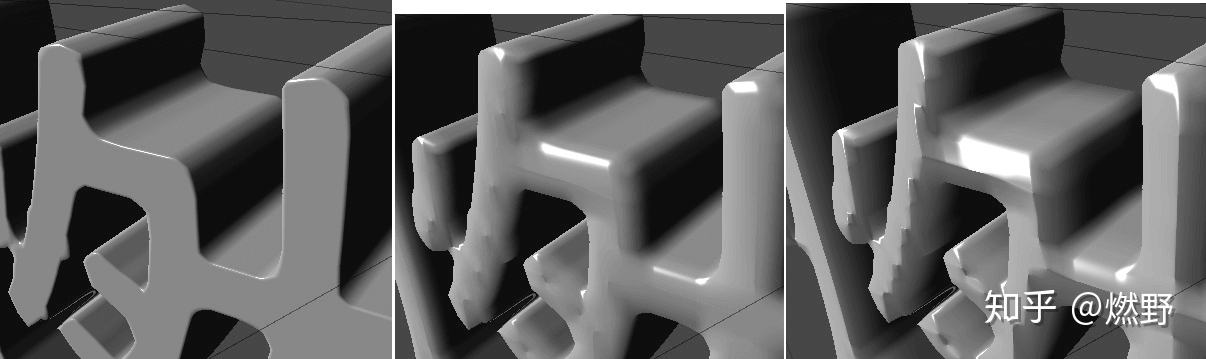## 三、表面纹理

``````// 采样face tex，可以是diffuse、normal、specular等
float4 SampleFaceTexByLocalPos(v2f input, float3 localPos, sampler2D tex)
{
float2 uv = localPos.xy * input.faceUvScaleOffset.xy + input.faceUvScaleOffset.zw;
// 所有face相关的tex都用这个tiling offset
uv = uv * _FaceDiffuseTex_ST.xy + _FaceDiffuseTex_ST.zw;
return tex2D(tex, uv);
}
``````

``````float4 SampleSideDiffuse(float3 localPos, float3 sideNormal)
{
// 左右面
float2 uvLR = localPos.yz * _LeftRightDiffuse_ST.xy + _LeftRightDiffuse_ST.zw;
float4 diffuseLR = tex2D(_LeftRightDiffuse, uvLR) * _LeftRightColor;

// 上下面
float2 uvUD = localPos.xz * _UpDownDiffuse_ST.xy + _UpDownDiffuse_ST.zw;
float4 diffuseUD = tex2D(_UpDownDiffuse, uvUD) * _UpDownColor;

sideNormal = abs(sideNormal);
// 非等比混合，UD会占得多
//return lerp(diffuseLR, diffuseUD, sideNormal.y);
// 等比混合
return lerp(diffuseLR, diffuseUD, sideNormal.y / (sideNormal.x + sideNormal.y));
}
``````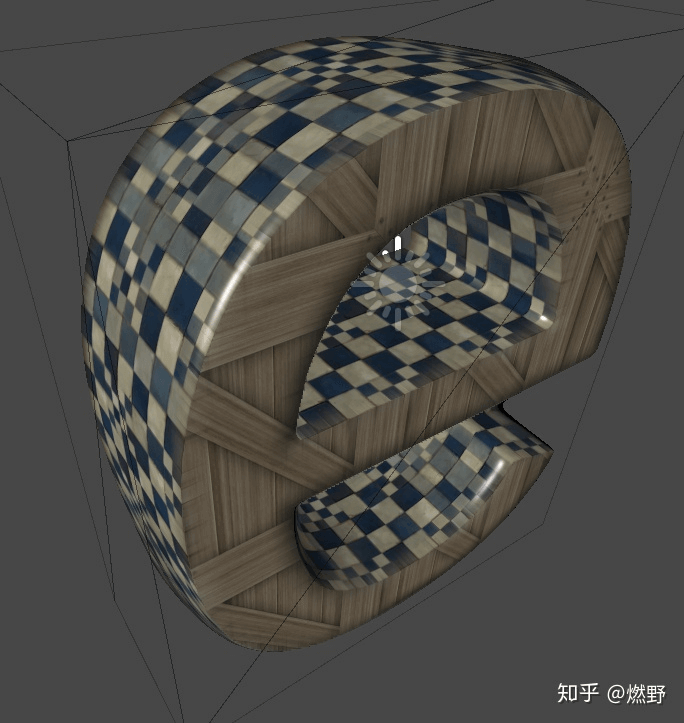triplanar diffuse mapping

6面纹理看起来还算不错，但是在正面和侧面边界有很硬的过渡，因为形状上还没做圆角过渡。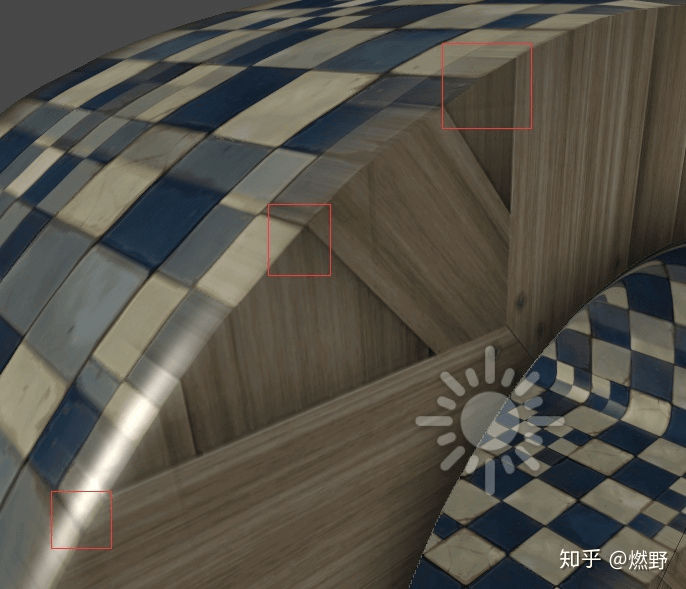## 四、形状的圆角过渡

IQ大神的这篇文章里有各种形状的SDF公式：
https://iquilezles.org/www/articles/distfunctions/distfunctions.html

``````Round Box - unsigned - exact
float udRoundBox( vec3 p, vec3 b, float r )
{
return length(max(abs(p)-b,0.0))-r;
}
``````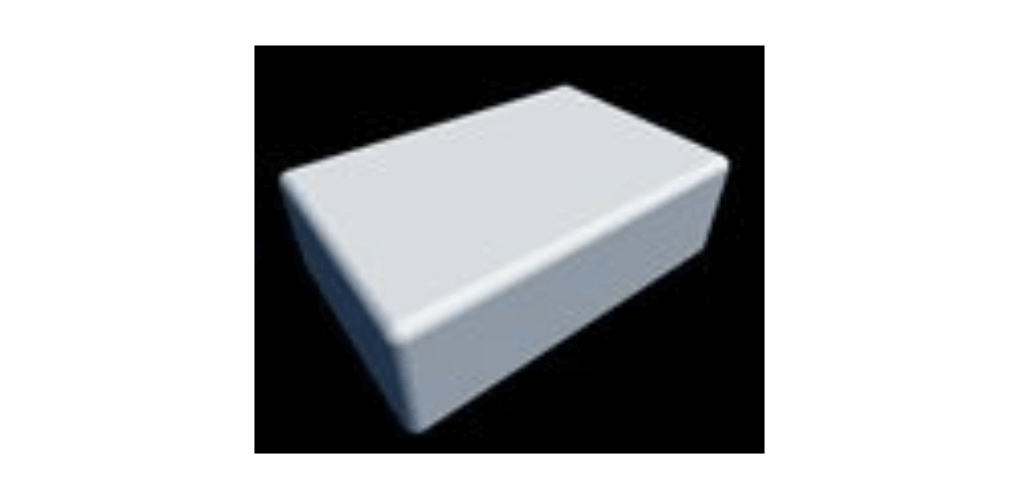A点都在两条边界外侧，A = (R, R - z) = (R, z到中间Z的距离-R到中间Z的距离）=

(R, abs(z - halfZ) - (halfZ - R))，这是为了支持反面圆角的情况。

B在x方向属于外侧，在z方向属于内侧。B = (R, R - z) = (R, abs(z - halfZ) - (halfZ - R))

C在x方向属于内侧，在z方向属于外侧，C = (-C.x到outline的距离, R) = ((_outline - sdfValue) * bound.w, R)

D都属于外侧，D = ((_outline - sdfValue) * bound.w, R)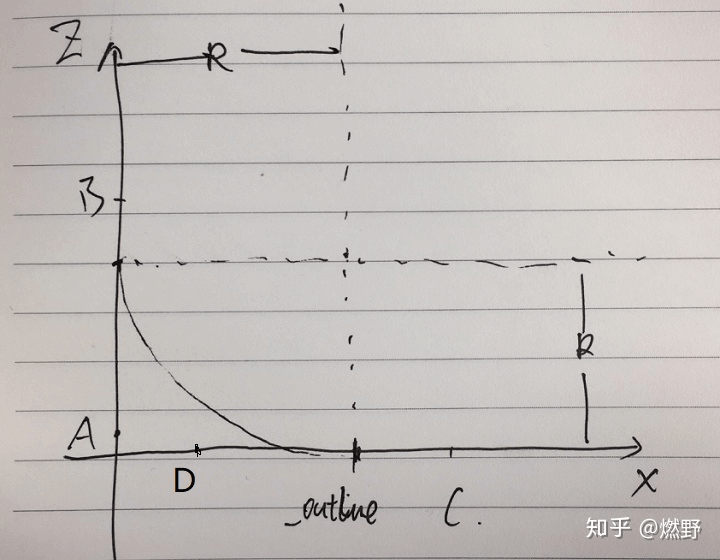``````// xz分量相对内退边界的距离，正表示在外，负表示在内
float2 meshOutlineDist = ((_outline - sdfValue) * input.bound.w + R, abs(localPos.z - halfZ)-(halfZ - R));
// length(max(meshOutlineDist, 0)) - R;就是对应上面udRoundBox公式求的值，表示3D空间下到形状的最近距离
``````

``````// 修改之前的检测，使支持形状的圆角过渡
float2 RayMarching(v2f input, out float3 localPos, float3 viewDir)
{
localPos = input.cuboidLocalPos.xyz;

// 用于剔除模型外顶点时微调
//float3 epsilon = input.bound.xyz * _boundEpsilon;
#if SDFRoundEdge
float halfZ = input.bound.z * 0.5;
#else
// 除以水平距离，这样在乘以水平距离就知道3d方向的增量
viewDir /= max(length(viewDir.xy), 0.001);
#endif

int i = 0;
float sdfValue;
for (; i < _loopCount; i++)
{
sdfValue = SampleSdfValueByLocalPos(input, localPos);
float sdfDist = _outline - sdfValue;
float meshDist;

#if SDFRoundEdge
// Round Box formula - unsigned - exact
//float udRoundBox(vec3 p, vec3 b, float r)
//{
//  return length(max(abs(p) - b, 0.0)) - r;
//}
float2 meshOutlineDist = float2(sdfDist * input.bound.w + radius, abs(localPos.z - halfZ) - (halfZ - radius));
meshDist = length(max(meshOutlineDist, 0)) - radius;
// 足够靠近边界就认为到达
if (meshDist <= 0)
return float2(i, sdfValue);
#else
// 足够靠近边界就认为到达
if (sdfDist <= _outlineEpsilon)
return float2(i, sdfValue);

meshDist = sdfDist * input.bound.w;
#endif
// sdf距离转到模型距离，向前march
localPos += viewDir * meshDist;

// 到达模型外面要被剔除掉
//clip((localPos + epsilon) * (input.bound.xyz - localPos + epsilon));
clip(localPos * (input.bound.xyz - localPos));
}

// 没有到达范围内，但应该也很接近了
sdfValue = SampleSdfValueByLocalPos(input, localPos);
return float2(i, sdfValue);
}
``````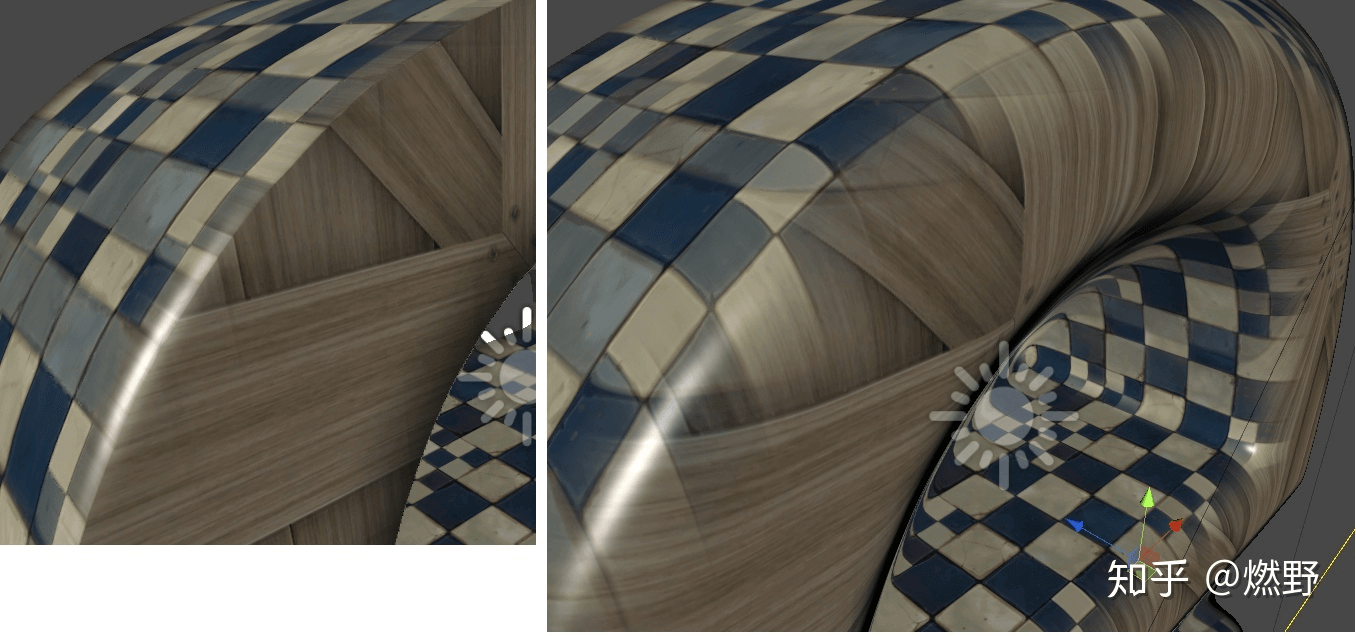## 五、法线纹理

https://catlikecoding.com/unity/tutorials/rendering/part-6

``````X.x * T + X.y * B + X.z * N;
// 简化一下，并且支持反面就是
float isForward = localPos.z > input.bound.z - localPos.z ? 1 : -1;
float3 finalNormal = X.xyz * float3(1, 1, isForward);
``````

``````X.x * T + X.y * B + X.z * N;
float isRight = sideNormal.x > 0 ? 1 : -1;
float3 leftRightNormal = X.zxy * float3(isRight, 1, 1);
``````

``````float isUp = sideNormal.y > 0 ? 1 : -1;
float3 upDownNormal = X.xzy * float3(1, isUp, 1);
``````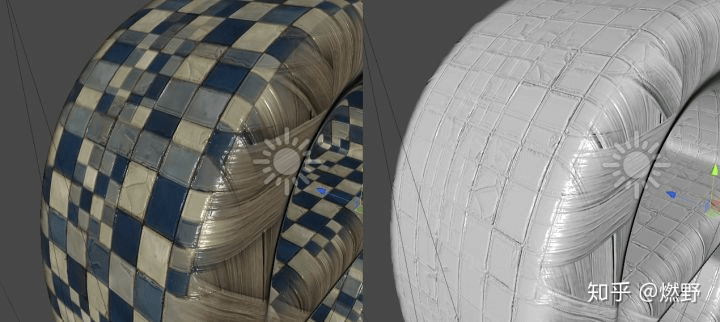## 六、其他问题

1、抗锯齿：由于MSAA是基于光栅化的，三角形边界并不是文字形状的边界，所以无效。基于后期的AA应该是可以的。还设想过识别文字形状周围的1像素做alpha混合来实现AA，外轮廓效果应该可以，如下图绿框中的。但就跟透明有类似的排序问题了，且文字之间的边缘原没法处理，如下图红框中的：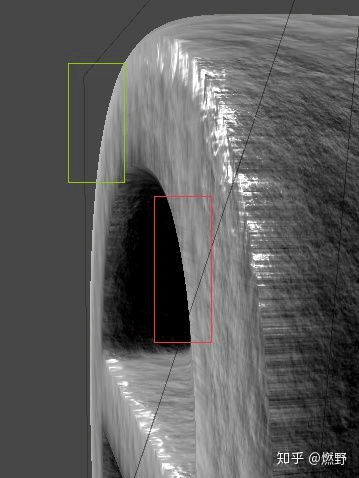2、遮挡：因为像素的深度值是文字Cube的边界，并不是模型的，一旦有模型穿插到文字体内会不正确，如下图，蓝色部分可见白色cube已经很接近文字cube的前面，但是红框中文字却没被遮挡。PS里写深度、RayMarching里每步测深度、调整渲染顺序应该能解决。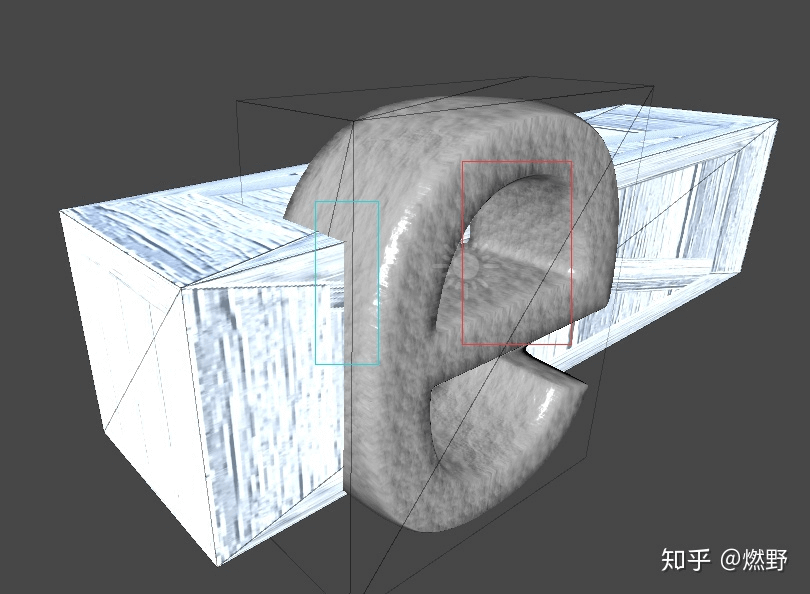4、字形过渡：SDF一大特性是能做一个形状到另一个形状的过渡，就是对SDF距离进行过渡，对于本例的文字来说要额外指定另一个字符的UV信息，如果文字Cube大小不一样也需要变化。

5、斜体：目前的机制没处理好斜体，如何变换到字符局部坐标系的问题。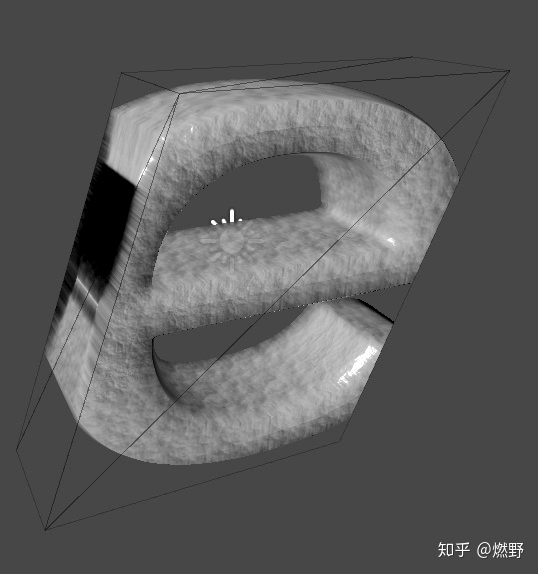6、下划线也不支持，还没研究TMP是怎么弄的。

QQ群：793972859（原群已满员）